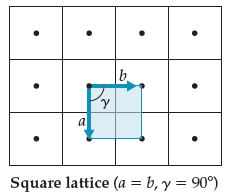# Problem: Consider the two-dimensional square lattice of the figure below. The "packing efficiency" for a two-dimensional structure would be the area of the atoms divided by the area of the unit cell, times 100%. What is the packing efficiency for a square lattice for atoms of radius large{frac {a}{2}} that are centered at the lattice points?

96% (36 ratings)
###### Problem Details

Consider the two-dimensional square lattice of the figure below. The "packing efficiency" for a two-dimensional structure would be the area of the atoms divided by the area of the unit cell, times 100%. What is the packing efficiency for a square lattice for atoms of radius that are centered at the lattice points?